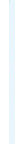## ISSN 2313-4607 (Online) ISSN 2304-8336 (Print)Home Editorial board For Authors Archive links SearchR. V. Boiko, D. I. Bilko, I. Z. Russu, N. M. Bilko

National University of Kyiv-Mohyla Academy, 2 G. Skovorody Str., Kyiv 04070

MATHEMATICAL ANALYSIS OF FUNCTIONAL PROPERTIES OF MICE BONE MARROW IN THE INITIAL PHASE OF ACUTE FRACTIONATED IRRADIATION

Objective: to determine the quantitative characteristics of population functioning of mice bone marrow colonyforming units during seven days of acute fractionated irradiation.
Materials and methods. Assigned task is solved by means of described in works R. V. Boiko et al. (2015, 2016) mathematical model of alterations in the number of bone marrow colony-forming units using the experimental results of work W.Chu-Tse, L. G.Lajtha (1975). Mathematical model is developed basing on the new hematopoiesis scheme, which was introduced by I. Chertkov(1984, 1991).
Results. By applying original mathematical model of hematopoiesis scheme using results concerning the change in number of bone marrow colony-forming units of mice femur we determined quantitative characteristics of their functioning during seven days of fractionated irradiation under daily acute ?-radiation in the dose of 0.7 Gy.
Conclusions. Mathematical model is introduced, which describes changes in the relative number of colony-forming units in mice bone marrow in the process of their acute fractionated irradiation.
Key words: ionizing radiation, bone marrow, functional properties, mathematical model.full text